# HBSE 7th Class Maths Solutions Chapter 9 Rational Numbers InText Questions

Haryana State Board HBSE 7th Class Maths Solutions Chapter 9 Rational Numbers InText Questions and Answers.

## Haryana Board 7th Class Maths Solutions Chapter 9 Rational Numbers InText Questions

Question 1.
Is the number $$\frac{2}{-3}$$ rational number ?
Solution:
$$\frac{2}{-3}$$ is a rational number.

Question 2.
List ten rational numbers.
Solution:
$$\frac{5}{2}, \frac{-3}{2}, 5,4,-1,-2, \frac{0}{1}, \frac{0}{-1}, \frac{0}{2}, \frac{-2}{3}$$ are rational numbers.Question 3.
Mention five rational numbers each of whose:
(a) Numerator is a negative integer and denominator is a positive integer.
(b) Numerator is positive integer and denominator is a negative integer.
(c) Numerator and denominator are both negative integers.
(d) Numerator and denominator are both positive integers.
Solution: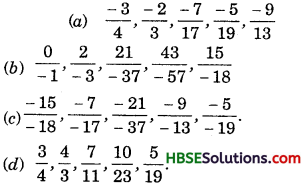Question 4.
Fill in the boxes: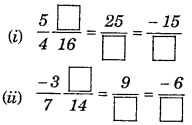Solution: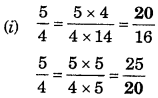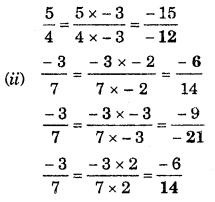Question 5.
Is 5 a positive rational number ?
Solution:
Yes, 5 is a positive rational number.Question 6.
List five more positive rational number.
Solution:
$$\frac{5}{6}, \frac{-7}{-11}, \frac{5}{7}, \frac{36}{63}, \frac{17}{19}$$

Question 7.
Q. 1. Is – 8 a negative rational number ?
Solution:
Yes, – 8 is a negative rational number.

Question 8.
List five more negative rational number.
Solution:
$$\frac{-16}{6}, \frac{-8}{3}, \frac{-24}{9}, \frac{-32}{12}, \frac{-40}{15}$$

Question 9.
Which of these are negative rational numbers ?
(i) $$\frac{-2}{3}$$
(ii) $$\frac{5}{7}$$
(iii) $$\frac{3}{-5}$$
(iv) 0
(v) $$\frac{6}{11}$$
(vi) $$\frac{-2}{-9}$$
Solution: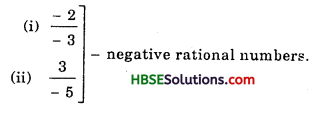Question 10.
Find the standard form of:
(i) $$\frac{-18}{45}$$
(ii) $$\frac{-12}{18}$$
Solution: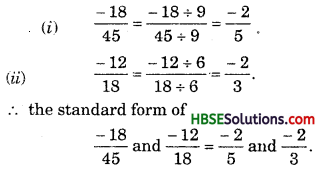Question 11.
Find five rational number between $$\frac{-5}{7}$$ and $$\frac{-3}{8}$$
Solution: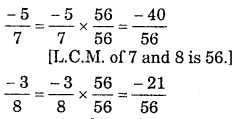Now we have common denominator. Number between – 40 and – 21 are – 39, -38, – 37, – 36, – 35, – 20.
So, we can choose any five out of them.

Question 12.
Find:
(i) $$\frac{-13}{7}+\frac{6}{7}$$
(ii) $$\frac{19}{5}+\frac{-7}{5}$$
Solution: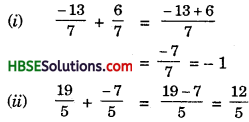Question 13.
Find:
(i) $$\frac{3}{7}+\frac{2}{3}$$
(ii) $$\frac{-5}{6}+\frac{-3}{11}$$
Solution: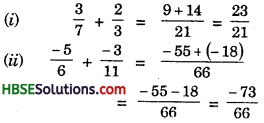Question 14.
(i) $$\frac{-3}{9}$$
(ii) $$\frac{-9}{11}$$ and
(iii) $$\frac{5}{7}$$
Solution:
(i) $$\frac{-3}{9}=\frac{3}{9}$$
(ii) $$\frac{-9}{11}=\frac{9}{11}$$
(iii) $$\frac{5}{7}=\frac{-5}{7}$$Question 15.
Find:
(i) $$\frac{7}{5}-\frac{2}{5}$$
(ii) $$2 \frac{1}{5}-\frac{-1}{3}$$
Solution: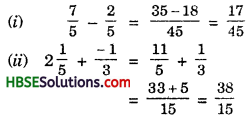Question 16.
What are the multiplication?
(i) $$\frac{-3}{4} \times 7$$
(ii) $$\frac{-6}{5} \times(-2)$$
Solution: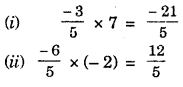Question 17.
Find:
(i) $$\frac{-3}{4} \times \frac{1}{7}$$
(ii) $$\frac{2}{3} \times \frac{-5}{9}$$
Solution:
(i) $$\frac{-3}{4} \times \frac{1}{7}=\frac{-3}{28}$$
(ii) $$\frac{2}{3} \times \frac{-5}{9}=\frac{-10}{27}$$

Question 18.
What will be the reciprocal of $$\frac{-6}{11}$$?$$\frac{-8}{5}$$?
Solution: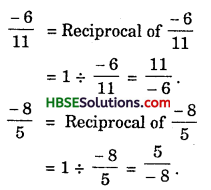Question 19.
Find
$$\frac{2}{3} \times \frac{-7}{8}$$
Solution:
$$\frac{2}{3} \times \frac{-7}{8}=\frac{-14}{24}=\frac{-7}{12}$$Question 20.
Find
$$\frac{-6}{7} \div \frac{5}{7}$$
Solution:
$$\frac{-6}{5} \div \frac{5}{7}=\frac{-6}{5} \times \frac{7}{5}=\frac{-42}{25}$$